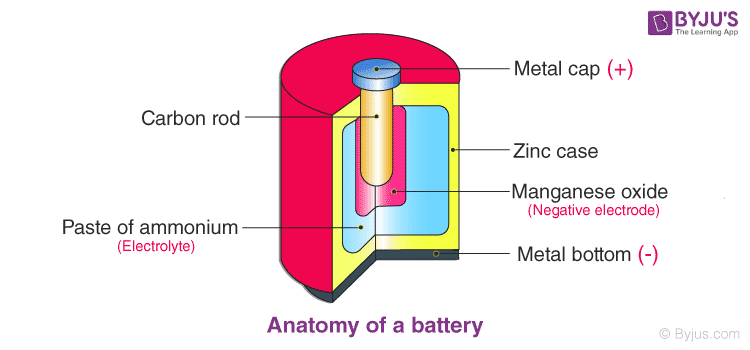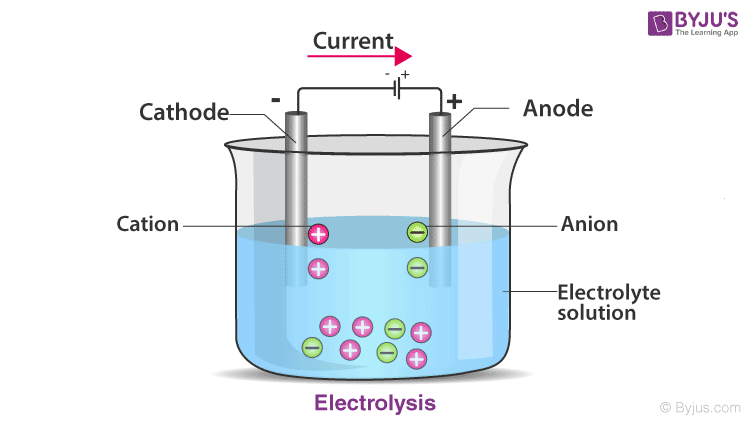# EMF: Cells, Electromotive Force And Internal Resistance

Batteries and cells are important inventions that have made a lot of our everyday tasks and life much easier. They are practically used in most of the portable electronic devices that we use today. Besides, we can say that we cannot imagine a world without them. In this article, let us know more about the cells and their working principle in detail.

## Cell or Electrochemical Cell

A cell or an electrochemical cell is a device that is capable of obtaining electrical energy from chemical reactions or vice versa. You have definitely seen a cell, the small AAA or AA batteries we use in our remotes.

An electric battery is a device made up of two or more cells that make use of the chemical energy stored in the chemicals and converts it into electrical energy. A battery is used to provide a continuous steady current source by way of providing constant EMF or Electromotive force to an electrical circuit or a machine.Each cell comprises two half-cells connected in series by a conductive electrolyte containing anions and cations. One half-cell is made up of the electrolyte and the negative electrode, the Anode. The negatively charged ions, also known as anions, migrate to the Anode. The other half-cell includes the electrolyte and the positive electrode, the Cathode, to which cations (positively charged ions) migrate.

Redox reactions, reduction and oxidation occur simultaneously and this powers the battery. Cations are reduced (it gains electrons) at the cathode during charging, while anions are oxidised (it loses electrons) at the anode during charging. During discharge, the process is reversed. The electrodes do not touch each other but are electrically connected by the electrolyte.## What Is an Electromotive Force (EMF) of a Cell?

When there is no electrical equipment attached to a cell i.e. no current is flowing through the cell, the electrolyte has the same potential throughout the cell. The condition of no current flowing through a cell is also known as an open circuit. In an open circuit, the potential of the cell becomes equal to the difference in the potentials of the electrodes. Anode has a positive potential (V+) whereas Cathode has a negative potential (-V). This potential difference is known as the Electromotive Force (EMF) of the cell and it is equal to;

$$\begin{array}{l}ξ = V_+ ~- ~(-V_-) = V_+~ + ~V_-\end{array}$$

We know that when we connect an appliance to the battery, a current flows through the circuit that is proportional to the voltage. The ratio of the voltage (V) across an object to the current flowing through it because of the potential is known as the resistance of that object. Resistance in Electricity can be linked to Friction in Mechanics. The electrical resistance of a body is the measure of the difficulty in passing an electric current through it. The SI unit of electrical resistance is ohm (Ω). The phenomenon of electrical resistance has led to the creation of electrical heaters and induction cooktops. Resistance is given by

$$\begin{array}{l}R = \frac{V}{I}\end{array}$$

The below video explains the relation between the EMF and voltage:### Internal Resistance

Similar to how a body opposes the flow of electricity through it, leading to resistance, the electrolytes in batteries have a finite value of resistance. The resistance generated inside a battery to the flow of current is referred to as the internal resistance of a battery (r). When a power source delivers current, the measured voltage output is lower than the voltage when no current is flowing through the circuit. This voltage drop is caused by the internal resistance of the battery to the flow of current through it.

#### Measuring Internal Resistance

The voltage of the battery can be measured using a voltmeter with no load connected to it. This is known as the open circuit voltage (VOC). VOC is equal to the voltage of the ideal voltage source in the battery. battery. If we connect a load across the battery, the voltage across the terminals drops. Let us look at an example to better understand this.

Measuring the current with a 8Ω external resistor between the poles of a 8 V battery, we would expect a current of the following value:

$$\begin{array}{l}I =\frac{V}{R} = 1 A \end{array}$$

In reality, when we measure we measure 0.8 A.

Hence the resistance is:

$$\begin{array}{l}R =\frac{V}{I} = 10 \, Ω \end{array}$$

So the internal resistance of the battery in this case is:

$$\begin{array}{l}R_{internal} = R_{total} – R_{external} \end{array}$$

Substiuting the values in the above equation, we get:

$$\begin{array}{l}R_{internal} = 10\, \Omega – 8\, \Omega = 2\, \Omega \end{array}$$

## Frequently Asked Questions – FAQs

Q1

### What is meant by electric potential?

Electric potential is the quantity of work required to displace a unit charge from a reference point to a specific point against an electric field.
Q2

### What is the full form of emf?

Electromotive force is the full form of emf.
Q3

### What is electromotive force?

Electromotive force or emf is equal to the potential terminal variation when no electric current flows.
Q4

### What is the unit of resistivity?

The unit of resistivity is given as ohm-metre.
Q5

### What is the reciprocal of resistance?

The reciprocal of resistivity is conductance.

Stay tuned to BYJU’S and Fall in Love with Learning!

Test your knowledge on Cells Electromotive Force And Internal Resistance